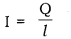# (a) Define the term ‘Coulomb’

(a) Define the term ‘Coulomb’.
(b) State the relationship between the electric current, the charge moving though a conductor and the time of flow.
Calculate the charge passing through an electric bulb in 20 minutes if the value of current is 200mA.

(a) Coulomb: Coulomb is the unit of electric charge. The amount of charge that passes through a conductor when 1 ampere current flows through the conductor for 1 coulomb. 1C = 1A × 1s

(b) If a net charge Q, flows across any cross-section of a conductor in time t, then the current/flowing through the cross section is:We have, Current flowing through the bulb, l = 200 mA = 0.2 A
Time of flow of current, t = 20min. = 1200s
Therefore, Q = l × t = 0.2 × 1200 = 240C
Hence, 240C charge is passed through the bulb.